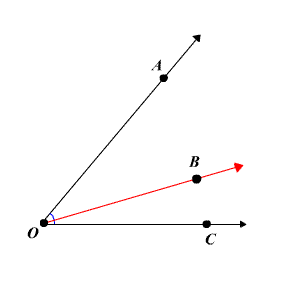The angle addition postulate states that if $B$ is in the interior of $AOC$ , then
$m\angle AOB+m\angle BOC=m\angle AOC$